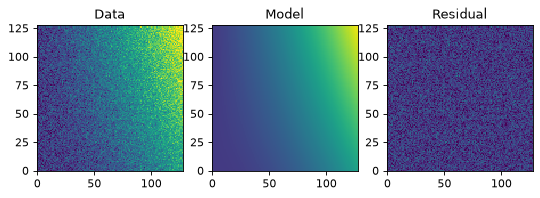# Fitting Models to Data¶

This module provides wrappers, called Fitters, around some Numpy and Scipy fitting functions. All Fitters can be called as functions. They take an instance of `FittableModel` as input and modify its `parameters` attribute. The idea is to make this extensible and allow users to easily add other fitters.

Linear fitting is done using Numpy’s `numpy.linalg.lstsq` function. There are currently two non-linear fitters which use `scipy.optimize.leastsq` and `scipy.optimize.fmin_slsqp`.

The rules for passing input to fitters are:

• Non-linear fitters currently work only with single models (not model sets).

• The linear fitter can fit a single input to multiple model sets creating multiple fitted models. This may require specifying the `model_set_axis` argument just as used when evaluating models; this may be required for the fitter to know how to broadcast the input data.

• The `LinearLSQFitter` currently works only with simple (not compound) models.

• The current fitters work only with models that have a single output (including bivariate functions such as `Chebyshev2D` but not compound models that map `x, y -> x', y'`).

## Simple 1-D model fitting¶

In this section, we look at a simple example of fitting a Gaussian to a simulated dataset. We use the `Gaussian1D` and `Trapezoid1D` models and the `LevMarLSQFitter` fitter to fit the data:

```import numpy as np
import matplotlib.pyplot as plt
from astropy.modeling import models, fitting

# Generate fake data
np.random.seed(0)
x = np.linspace(-5., 5., 200)
y = 3 * np.exp(-0.5 * (x - 1.3)**2 / 0.8**2)
y += np.random.normal(0., 0.2, x.shape)

# Fit the data using a box model.
# Bounds are not really needed but included here to demonstrate usage.
t_init = models.Trapezoid1D(amplitude=1., x_0=0., width=1., slope=0.5,
bounds={"x_0": (-5., 5.)})
fit_t = fitting.LevMarLSQFitter()
t = fit_t(t_init, x, y)

# Fit the data using a Gaussian
g_init = models.Gaussian1D(amplitude=1., mean=0, stddev=1.)
fit_g = fitting.LevMarLSQFitter()
g = fit_g(g_init, x, y)

# Plot the data with the best-fit model
plt.figure(figsize=(8,5))
plt.plot(x, y, 'ko')
plt.plot(x, t(x), label='Trapezoid')
plt.plot(x, g(x), label='Gaussian')
plt.xlabel('Position')
plt.ylabel('Flux')
plt.legend(loc=2)
```

(png, svg, pdf)As shown above, once instantiated, the fitter class can be used as a function that takes the initial model (`t_init` or `g_init`) and the data values (`x` and `y`), and returns a fitted model (`t` or `g`).

## Simple 2-D model fitting¶

Similarly to the 1-D example, we can create a simulated 2-D data dataset, and fit a polynomial model to it. This could be used for example to fit the background in an image.

```import warnings
import numpy as np
import matplotlib.pyplot as plt
from astropy.modeling import models, fitting

# Generate fake data
np.random.seed(0)
y, x = np.mgrid[:128, :128]
z = 2. * x ** 2 - 0.5 * x ** 2 + 1.5 * x * y - 1.
z += np.random.normal(0., 0.1, z.shape) * 50000.

# Fit the data using astropy.modeling
p_init = models.Polynomial2D(degree=2)
fit_p = fitting.LevMarLSQFitter()

with warnings.catch_warnings():
# Ignore model linearity warning from the fitter
warnings.simplefilter('ignore')
p = fit_p(p_init, x, y, z)

# Plot the data with the best-fit model
plt.figure(figsize=(8, 2.5))
plt.subplot(1, 3, 1)
plt.imshow(z, origin='lower', interpolation='nearest', vmin=-1e4, vmax=5e4)
plt.title("Data")
plt.subplot(1, 3, 2)
plt.imshow(p(x, y), origin='lower', interpolation='nearest', vmin=-1e4,
vmax=5e4)
plt.title("Model")
plt.subplot(1, 3, 3)
plt.imshow(z - p(x, y), origin='lower', interpolation='nearest', vmin=-1e4,
vmax=5e4)
plt.title("Residual")
```

(png, svg, pdf)